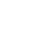Frontpage Search Email . Danish .Welcome to my homepage, developing the classic head physicsMass attraction. Mass attraction is mass. To see the details of this here, read my section about mass formation. There are two types of mass attraction: Mass-attraction of massive mass (particle mass). Formula for rest mass is mx = Vx * c2 and formula for mass attraction is m/s = (Vx * c2) / V. The cutting point is the delimited periphery of the mass and transition to the normal function of the universe, after which the attraction is decreasing. This is a ball formula, which assumes that the mass is at rest. If the mass is not at rest, there shall be coordinated for the fundamental functions of the universe.Molecular mass attraction (the gravity). Formula of molecular mass attraction is m/s = (Vx * v2) / V The cutting point is the same as in mass attraction. Example: The earth's cutting point is the surface and v = 9.8  m /s. This is also a ball formula, which assumes that the Earth is at rest. If the Earth is not at rest, there shall be coordinated for the fundamental functions of the universe, if it all shall be correct.The difference between the two systems is the combinatorial composition. Mass attraction is that part of the universe which holds the various particle parts together. Example: If we take the earth that is made up of molecules and would like to calculate what the mass attraction is at a height of 50 km above the surface: The formula is m/s = (Vx * v2) / V Gravity is 9.8 m / s The radius of the earth is 6,371 km equals 6 371 000 m. The distance is 6,371 km + 50 km is = 6 371 000 m + 50 000 m = 6 421 000 m Gravity is at 50 km altitude: m / s = ((4/3 * pi * (6 371 000)3 ) * 9.8) / (4/3 * pi * (6 421 000)3 ) = 9.57 m / s The universe's two main parameters are volume and velocity, if you remove the volume parameter and replace it with a charge system, then the error is so great that it cannot be greater, all the basic systems will appear as an incoherent incomprehensible chaos.Classic big bang. Mass formation. Atom structure. Atom binding. Molecular binding. Particle radiation Magnetic fields. Mass attraction. Conclusion. Micro physicsUltima update :  1 september 2019
Email : info@jwhdk.eu